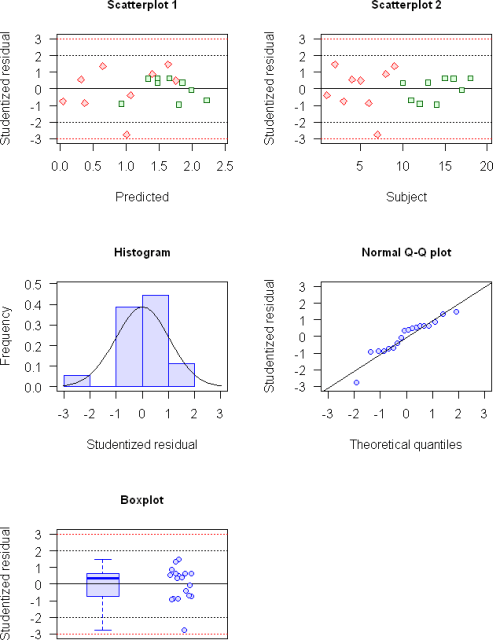## Phoenix → R [General Sta­tis­tics]

Hi Lucas,

export the stuff toand some quick/dirty code:
In the Phoenix-project navigate to Stud Res intra > Output Data > Result right-click > Export
Filename: StudResIntra Filetype: Text (Tab delimied) (*.txt) Save
The default location is the desktop (which conveniently is also the default import site in R). Start R and paste this code into the console:

res <- read.table("StudResIntra.txt", header=TRUE, sep="\t") attach(res) ResLim <- c(-max(abs(StudResIntra))*1.1, max(abs(StudResIntra))*1.1) PreLim <- c(min(abs(Predicted))*1.1, max(abs(Predicted))*1.1) SubLim <- c(min(abs(Subject))*1.1, max(abs(Subject))*1.1) TR <- subset(res, Sequence == "TR") RT <- subset(res, Sequence == "RT") RL <- function() {   abline(h=c(0, -2, 2, -3, 3), lty=c(1, rep(2, 4)),     col=c(rep("black", 2), rep("red", 2))) } lab <- "Studentized residual" split.screen(c(3, 2)) screen(1) plot(0, 0, type="n", xlim=PreLim, ylim=ResLim, xlab="Predicted", ylab=lab,   main="Scatterplot 1", cex.main=0.9, las=1) RL() points(TR$Predicted, TR$StudResIntra, pch=23, col="red", bg="#ffdddd") points(RT$Predicted, RT$StudResIntra, pch=22, col="darkgreen", bg="#ddffdd") screen(2) plot(0, 0, type="n", xlim=SubLim, ylim=ResLim, xlab="Subject", ylab=lab,   main="Scatterplot 2", cex.main=0.9, las=1) RL() points(TR$Subject, TR$StudResIntra, pch=23, col="red", bg="#ffdddd") points(RT$Subject, RT$StudResIntra, pch=22, col="darkgreen", bg="#ddffdd") screen(3) HisLim <- c(0, max(hist(StudResIntra, breaks="FD", plot=FALSE)\$density)*1.1) hist(StudResIntra, breaks="FD", freq=FALSE, xlim=ResLim, ylim=HisLim, xlab=lab,   ylab="Frequency", main="Histogram", cex.main=0.9, las=1, border="blue",   col="#ddddff") curve(dnorm(x, mean=mean(StudResIntra), sd=sd(StudResIntra)), add=TRUE) box() screen(4) qqnorm(StudResIntra, xlim=ResLim, ylim=ResLim, xlab="Theoretical quantiles",   ylab=lab, main="Normal Q-Q plot", cex.main=0.9, pch=21, col="blue", bg="#ddddff",   las=1, plot.it=TRUE) qqline(StudResIntra, datax=F, distribution=qnorm) screen(5) boxplot(StudResIntra, xlim=c(0.5, 2.5), ylim=ResLim, ylab=lab, main="Boxplot",   cex.main=0.9, las=1, at=1, border="blue", col="#ddddff") RL() points(x=jitter(rep(2, length(StudResIntra)), factor=4), StudResIntra,   pch=21, col="blue", bg="#ddddff") close.screen(all = TRUE) detach(res)Good luck! If you have a Connect license you even don’t have to leave PHX.

Dif-tor heh smusma 🖖
Helmut SchützThe quality of responses received is directly proportional to the quality of the question asked. 🚮
Science QuotesIng. Helmut Schütz